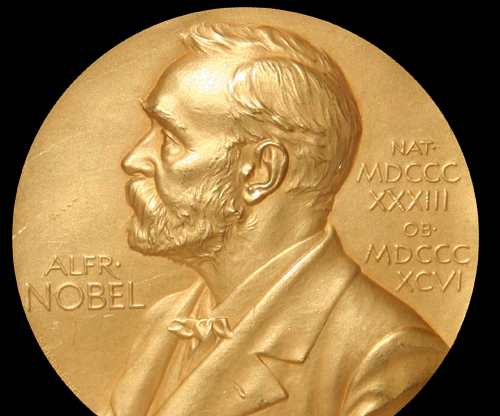# What Is the Zeeman Effect?

Ray Hawk
Ray HawkThe Zeeman Effect is named after Pieter Zeeman, a physicist from the Netherlands who won the Nobel Prize in Physics along with Hendrik Lorentz in 1902 for discovering it.

The Zeeman effect is a property in physics where the light of a spectral line is split into two or more frequencies when under the presence of a magnetic field. The property is named after Pieter Zeeman, a 20th century physicist from the Netherlands who won the Nobel Prize in Physics along with Hendrik Lorentz in 1902, for discovering the effect. The development of quantum mechanics further modified understanding of the Zeeman effect by determining which spectral lines were emitted as electrons were moved from one energy shell to another in their orbit of atomic nuclei. Understanding of the Zeeman effect has led to advancement in electron paramagnetic resonance studies, as well as the measurement of magnetic fields in space such as those of the Sun and other stars.

Contemplating how the Zeeman effect in hydrogen takes place is one of the easiest methods of understanding the process. A magnetic field applied to a hydrogen transition spectral line will cause an interaction with the magnetic dipole moment of orbital angular momentum for the electron and split the spectral line into three lines. Without the magnetic field, spectral emission is in a single wavelength, which is governed by principal quantum numbers.

The Zeeman effect can also be divided into the anomalous Zeeman effect and the normal Zeeman effect. The normal Zeman effect is characterized by such atoms as hydrogen, where an expected transition into an equally-spaced display of a triplet of spectral lines occurs. In an anomalous effect, the magnetic field may instead split the spectral lines into four, six, or more divisions, with wider-than-expected spacings between the wavelengths. The anomalous effect deepened the understanding of electron spin, and is something of a mislabel, as it is now a predicted effect.

The experimental results of studying this phenomenon concluded that the spin state, or orientation of the electron, was key to the energy change it underwent and, therefore, the type of spectral emission it produced. If the plane of orbit for an electron was perpendicular to an applied magnetic field, then it would produce a positive or negative energy change state depending on its rotation. If the electron was within the plane of its orbit around the nucleus, the net force or energy change state would be zero. This concluded that Zeeman splitting effects could be calculated based on the orbit, or angular momentum of an electron, relative to any applied magnetic field.

Original observations suggested that the normal Zeeman effect witnessed with hydrogen, where a split to three spectral lines occurred, would be common. In reality, this turned out to be an exception to the rule, however. This is because the three spectral line split is based on angular momentum, or orbit of an electron around the nucleus, yet an electron spin state has twice the magnetic moment of angular momentum. Spin state is seen as a larger factor, therefore, in producing the Zeeman effect, and spin states, or electron rotations, have to be theoretically predicted using quantum electrodynamics.

## You might also Like